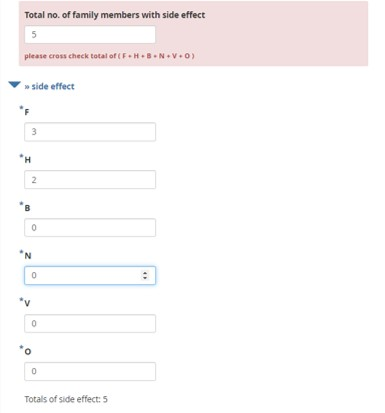# The sum of 5 questions should be equal to the input made for the 6th question

Hiii,
How to validate a group of 5 questions if sum of 5 questions are not equal to sixth question I. e.
Total Population = q1+q2+q3+q4+q5
Looking for your help.

@dantd

Kindly add a formula that combines both if function and sum as a constraint that you want.

Welcome to the community @dantd! Do you mean you wish to make a sum from q1 to q5 and then see if the sum is equal to q6 or not? Did not quite understand your issue. Could you kindly simplify it so that the community could help you solve your issue.

Thanks for the reply, I tried but could not work…

@Kal_Lam, thanks for the reply, actually i am having total 6 questions, where q1 to q5 and the sum of all 5 questions must be equal to question 6, if not equal then could not move ahead.
Tried to validate same with each question but not worked, then tried to calculate question, but there is no validation available at calculated question.

I think it is now clear…

Sorry for my bad explanation…

@dantd, you could do it as outlined in the image shared below:

#### Reference xlsform:

Constaint.xlsx (9.8 KB)

@Kal_Lam thanks it is exactly what i wanted, but its not working in my form as i am using below formula for validation
.=coalesce(\${F},0)+coalesce(\${H},0)+coalesce(\${B},0)+coalesce(\${N},0)+coalesce(\${V},0)+coalesce(\${O},0)

is there any error in above formula, please go through my form response too…Use the `constraint` and the `constraint message` in the last question.